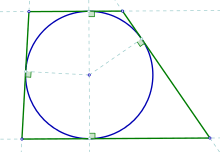# Tangential polygonA tangential trapezoid

In Euclidean geometry, a tangential polygon, also known as a circumscribed polygon, is a convex polygon that contains an inscribed circle (also called an incircle). This is a circle that is tangent to each of the polygon's sides. The dual polygon of a tangential polygon is a cyclic polygon, which has a circumscribed circle passing through each of its vertices.

All triangles are tangential, as are all regular polygons with any number of sides. A well-studied group of tangential polygons are the tangential quadrilaterals, which include the rhombi and kites.

## Characterizations

A convex polygon has an incircle if and only if all of its internal angle bisectors are concurrent. This common point is the incenter (the center of the incircle).

There exists a tangential polygon of n sequential sides a1, ..., an if and only if the system of equationshas a solution (x1, ..., xn) in positive reals. If such a solution exists, then x1, ..., xn are the tangent lengths of the polygon (the lengths from the vertices to the points where the incircle is tangent to the sides).

## Uniqueness and non-uniqueness

If the number n of sides is odd, then for any given set of sidelengthssatisfying the existence criterion above there is only one tangential polygon. But if n is even there are an infinitude of them.:p. 389 For example, in the quadrilateral case where all sides are equal we can have a rhombus with any value of the acute angles, and all rhombi are tangential to an incircle.

If the n sides of a tangential polygon are a1, ..., an, the inradius (radius of the incircle) iswhere K is the area of the polygon and s is the semiperimeter. (Since all triangles are tangential, this formula applies to all triangles.)

## Other properties

• For a tangential polygon with an odd number of sides, all sides are equal if and only if all angles are equal (so the polygon is regular). A tangential polygon with an even number of sides has all sides equal if and only if the alternate angles are equal (that is, angles A, C, E, ... are equal, and angles B, D, F, ... are equal).
• In a tangential polygon with an even number of sides, the sum of the odd numbered sides' lengths is equal to the sum of the even numbered sides' lengths.
• A tangential polygon has a smaller area than any other polygon with the same perimeter and the same interior angles in the same sequence.:p. 862
• The centroid of any tangential polygon, the centroid of its boundary points, and the center of the inscribed circle are collinear, with the polygon's centroid between the others and twice as far from the incenter as from the boundary's centroid.:pp. 858–9

## Tangential triangle

While all triangles are tangential to some circle, a triangle is called the tangential triangle of a reference triangle if the tangencies of the tangential triangle with the circle are also the vertices of the reference triangle.